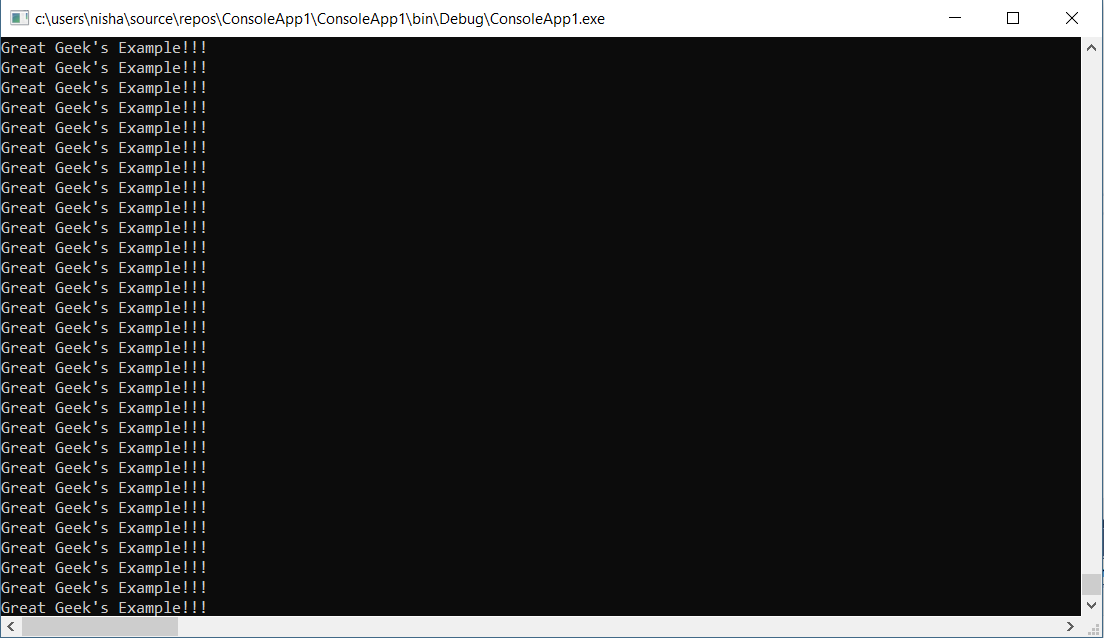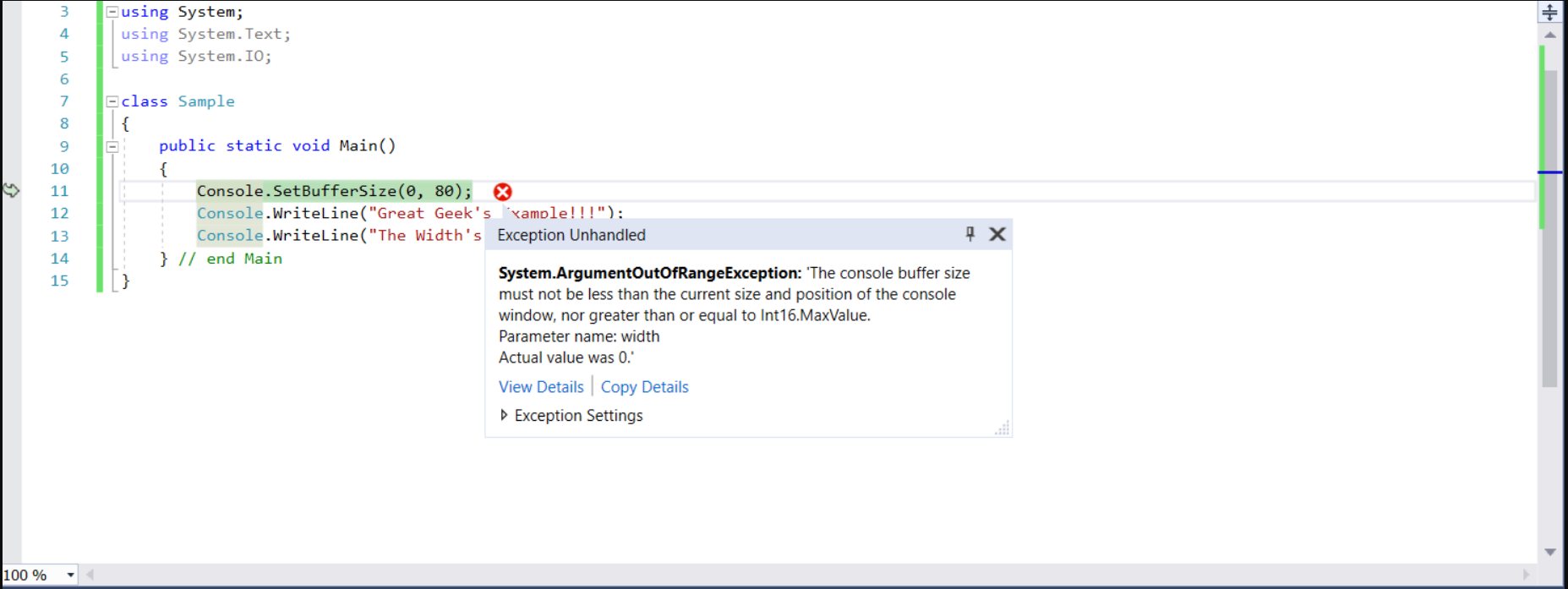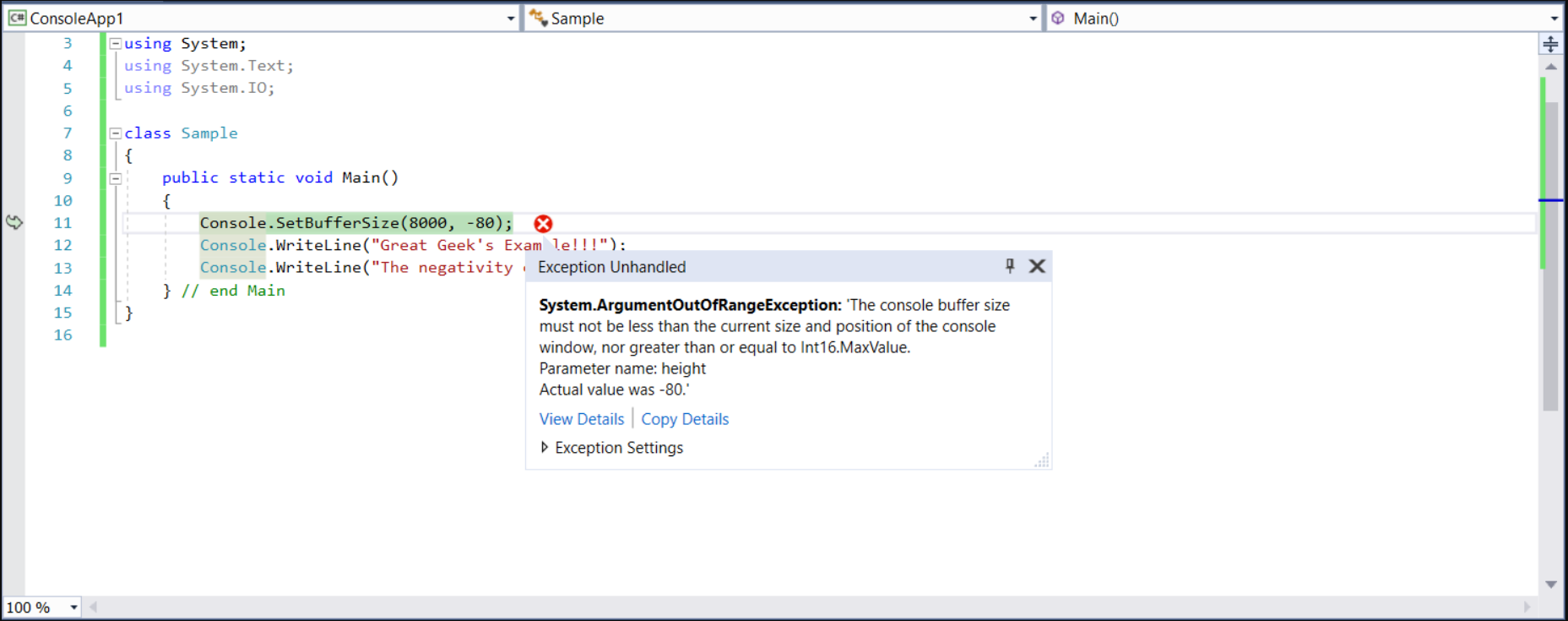Related Articles
Console.SetBufferSize() Method in C#
• Last Updated : 14 Mar, 2019

Console.SetBufferSize(Int32, Int32) Method is used to set the height and width of the screen buffer area to the specified values.

Syntax: public static void SetBufferSize(int width, int height);

Parameters:
width: It sets the width of the buffer area measured in the form of columns.
height: It sets the height of the buffer area measured in the form of rows.

Return value: The new size of the buffer screen.

Exceptions:

• ArgumentOutOfRangeException: If the height or width is less than or equal to zero Or height or width is greater than or equal to MaxValue. Also, if the width is less than `WindowLeft + WindowWidth` or height is less than `WindowTop + WindowHeight` then we will get the same exception.
• IOException: If an I/O error occurred.

Note: As you will see via the horizontal and the vertical scrollbars in the below examples, as we give different dimensions, we get differently sized windows.

Example 1:

 `// C# program to demonstrate ` `// the  SetBufferSize Method ` `using` `System; ` `using` `System.Text; ` `using` `System.IO; ` ` `  `class` `GFG { ` ` `  `    ``// Main Method ` `    ``public` `static` `void` `Main() ` `    ``{ ` ` `  `        ``// using the method ` `        ``Console.SetBufferSize(800, 800); ` `        ``Console.WriteLine(``"Start"``); ` `        ``while` `(``true``)  ` `        ``{ ` `            ``Console.WriteLine(``"Great Geek's Example!!!"``); ` `        ``} ` `    ``} ``// end Main ` `} `

Output:Example 2:

 `// C# program to demonstrate ` `// the SetBufferSize Method ` `using` `System; ` `using` `System.Text; ` `using` `System.IO; ` ` `  `class` `GFG { ` ` `  `    ``// Main Method ` `    ``public` `static` `void` `Main() ` `    ``{ ` `        ``Console.SetBufferSize(0, 80); ` `        ``Console.WriteLine(``"Great Geek's Example!!!"``); ` `        ``Console.WriteLine(``"The Width's value is too less!"``); ` `    ``} ``// end Main ` `} `Example 3:

 `// C# program to demonstrate ` `// the SetBufferSize Method ` `using` `System; ` `using` `System.Text; ` `using` `System.IO; ` ` `  `class` `GFG { ` ` `  `    ``// Main Method ` `    ``public` `static` `void` `Main() ` `    ``{ ` `        ``Console.SetBufferSize(8000, -80); ` `        ``Console.WriteLine(``"Great Geek's Example!!!"``); ` `        ``Console.WriteLine(``"The negativity of this height is unbearable!"``); ` `    ``} ``// end Main ` `} `Reference:

My Personal Notes arrow_drop_up
Recommended Articles
Page :